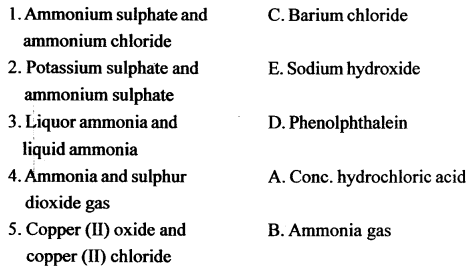# New Simplified Chemistry Class 10 ICSE Solutions – Study Of Compounds : Ammonia

## New Simplified Chemistry Class 10 ICSE Solutions – Study Of Compounds: Ammonia

Viraf J Dalal Chemistry Class 10 Solutions and Answers

Questions
2001

Question 1.
State what do you observe when :
Neutral litmus solution is added to an alkaline solution.
The litmus solution turns to blue.

Question 2.
Name (formula is not acceptable) the gas produced in the following reaction :
Warming ammonium sulphate with sodium hydroxide solution.
Ammonia gas.

Question 3.
Write the equation for the preparation of NH3 from ammonium chloride and calcium hydroxide.
2NH4Cl + Ca(OH)2 → CaCl2 + 2NH3 + 2H2O

Question 4.
What are the products formed when ammonia is oxidized with copper oxide.2002

Question 1.
From the following gases – ammonia, chlorine, hydrogen chloride, sulphur dioxide, select the gas that turns moist red litmus paper blue.
Write the equation for the reaction – when the gas is passed over heated CuO.
Ammonia gas.
3CuO + 2NH3 → 3Cu + N2 + 3H2O

2003

Question 1.
Name a gas whose solution in water is alkaline.
Ammonia

Question 2.
How would you distinguish between Zn2+ and Pb2+ using ammonium hydroxide solution.
Zinc salt gives white gelatinous ppt. and Pb2+ gives chalky white ppt. with ammonium hydroxide.

Question 3.
Write the equation for the formation of ammonia by the action of water on mangesium nitride.
Mg3N2 + 6H2O → 2NH3 + 3Mg (OH)2

Question 4.
How is ammonia collected. Why is ammonia not collected over water.
Ammonia gas is lighter than air and hence collected by the downward displacement of air.
Ammonia is not collected over water because it is highly soluble in water.

Question 5.
Which compound is normally used as a drying agent for ammonia.
Quicklime(Calcium oxide CaO).

2004

Question 1.
From the gases ammonia, hydrogen chloride, hydrogen sulphide, sulphur dioxide – Select the following :

1. When this gas is bubbled through copper sulphate soln., a deep blue coloured solution is formed.
2. This gas burns in oxygen with a green flame.

1. ammonia
2. ammonia

Question 2.
Write the equation for the reaction in the Haber’s process that forms ammonia.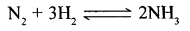Question 3.
State the purpose of liquefying the ammonia produced in the process.
By liquefying ammonia, it can be easily seperated from unreacted N2 and H2 gases.

Question 4.
Write an equation for the reaction of chlorine with excess of ammonia.2005

Question 1.
Name the ion other than ammonium ion formed when ammonia dissolves in water.
Hydroxyl ion (NH3 + H2O → NH++ OH )

Question 2.
Write the equations for the following reactions which result in the formation of ammonia.

1. A mixture of ammonium chloride and slaked lime is heated.
2. Aluminium nitride and water.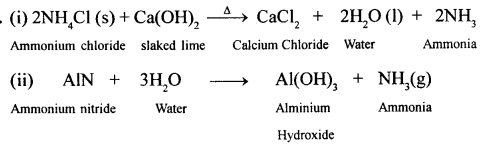2006

Question 1.
Select the correct compound from the list given — Ammonia, Copper oxide, Copper sulphate, Hydrogen chloride, Hydrogen sulphide, Lead bromide — which matches the description given below :
The compound is not a metal hydroxide, its aqueous solution is alkaline in nature.
Ammonia.

Question 2.
From the list of substances given — Ammonium sulphate, Lead carbonate, Chlorine, Copper nitrate, Ferrous sulphate — State :
A compound which on heating with sodium hydroxide produces a gas which forms dense white fumes with hydrogen chloride.
Ammonium sulphate.

Question 3.
State what is observed when excess of ammonia is passed through an aq. solution of lead nitrate.
Chalky white insoluble ppt. is formed.

Question 4.
Name the substance used for drying ammonia,
Quick lime (CaO).

Question 5.
Write a balanced chemical equation to illustrate the reducing nature of ammonia.
Reducing nature of ammonia.
2NH3 + 3CuO → 3Cu + 3H2 + N2 (g)

Question 6.
With reference to Haber’s process for the preparation of ammonia, write the equation and the conditions required.Conditions for maximum yield of ammonia

1. High Pressure (700-900 atm)
2. Optimum temperature (450-500°C)
3. Use of a catalyst : Iron (Fe) and use of a promotor : Molybdenum (Mo)

2007

Question 1.
Write a balanced equation for the following reaction :
Ammonium sulphate from ammonia and dilute sulphuric acid.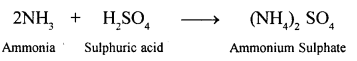Question 2.
Give equation for – reaction in which NH3 is oxidized by :

1. a metal oxide ;
2. a gas which is not oxygen.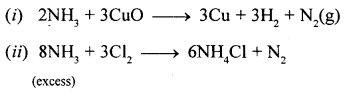Question 3.
You enter a laboratory after a class has completed the Fountain Experiment. How will you be able to tell whether the gas used in the experiment was hydrogen chloride or ammonia.
If the colour of the litmus solution in the round bottomed flask is blue the gas used was ammonia and if the colour of the litmus solution in the round bottomed flask is red, the gas used was hydrogen chloride. This is because hydrogen chloride(aq) is acidic in nature and turns purple litmus solution red and ammonia (aq) is basic in nature and turns purple neutral litmus solution blue.

2008

Question 1.
Ammonia can be obtained by adding water to : [Select the correct word]
A Ammonium chloride
B Ammonium nitrite
C Magnesium nitride
D Magnesium nitrate
C Magnesium nitride

Question 2.
Name : An alkaline gas A which gives dense white fumes with hydrogen chloride.
NH3 (Ammonia)

Question 3.
Write the equation for the following reaction : Aluminium nitride and water.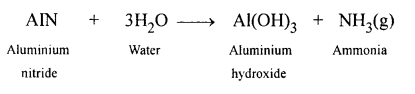Question 4.
Complete the table relating to an important industrial processes. [Output refers to the product of the process].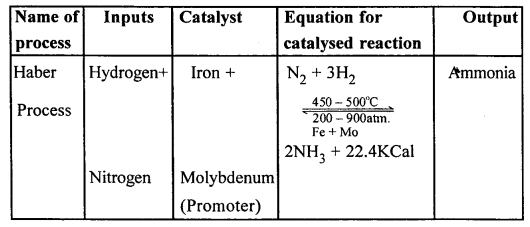2009

Question 1.
Name the gas – that burns in oxygen with a green flame.
Ammonia (NH3)

Question 2.
Write a fully balanced equation for the following : Magnesium nitride is treated with warm water.Question 3.
Identity the substances ‘Q’ based on the information given :
The white crystalline solid Q is soluble in water, it liberates a pungent smelling gas when heated with sodium hydroxide solution.
Q is Ammonium chloride and pungent smelling gas is ammonia.

2010

Question 1.
Complete the blanks (a) to (e) in the passage given, using the following words.
(Ammonium, reddish brown, hydroxyl, nitrogen dioxide, ammonia, dirty green, alkaline, acidic) in the presence of a catalyst, nitrogen and hydrogen combine
to give (a) _____ gas. When the same gas is passed through water, it forms a solution which will be _____ (b) in nature, and will contain the ions (c) _____ and (d) _____ (e) _____ coloured precipitate of iron [II] hydroxide is formed when the above solution is added to iron [II] sulphate solution, sssssss

(a) Ammonia
(b) Alkaline
(c) Ammonium
(d) Hydroxyl
(e) Dirty green

Question 2.
State your observation when – in the absence of a catalyst ammonia gas is burnt in an atmosphere of oxygen.
Greenish yellow flame is observed.

Question 3.
Give the equation for the reaction : ammonium chloride is heated with sodium hydroxide.
NH4Cl + NaOH → NaCl + NH3 + H2O

Question 4.
In the manufacture of ammonia.

1. Name the process.
2. State the ratio must the reactants taken ?
3. State the catalyst used.
4. Give the equation for the manufacture of the gas ammonia.Question 5.
Write a relevant equation, to show that ammonia can act as a reducing agent
2NH3 + 3Cl2 → N2 + 6HCl

Question 6.
Name two gases you have which can be used to study the fountain experiment.
State the common property demonstrated by the fountain experiment ?
Two gases which are used to study the fountain experiment are Hydrogen chloride gas (HCl) and Ammonia (NH3)
The common property of these two gases demonstrated by the fountain experiment is the solubility of gases

2011

Question 1.
State what is observed when – Ammonium hydroxide is first added in a small quantity and then in excess to a solution of copper sulphate.
On adding ammonium hydroxide in small amount, pale blue precipitates will appear. On adding ammonium hydroxide in excess, blue precipitates will dissolve forming deep blue solution.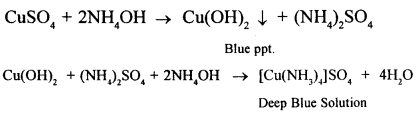Question 2.
The diagram shows set up for the laboratory preparation of a pungent alkaline gas.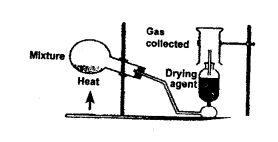1. Name the gas collected in the jar.
2. Write the balanced equation for the above preparation
3. State how the gas is collected?
4. Name the drying agent used.
5. State how you will find out that the jar is full of pungent gas?

1. Ammonia (NH3)
2. NH4 Cl + Ca (OH)2 → CaCl2 + 2H2O + 2NH3
3. Downward displacement of air
4. Quicklime (CaO)
5. Bring a rod dipped in HCl near it. Dense white fumes of ammonium chloride will be formed.

Question 3.
Write a balanced chemical equation – Chlorine reacts with excess of ammonia.Question 4.
State your observation when – Water is added to the product formed, when aluminium is burnt in a jar of nitrogen gas.
Pungent smelling and alkaline gas (NH3) is evolved.2012

Question 1.
Name the gas produced when excess ammonia reacts with chlorine.
Nitrogen.

Question 2.
Rewrite the correct statement with the missing word/s Magnesium nitride reacts with water to liberate ammonia.
Magnesium nitride reacts with warm water to liberate ammonia along with magnesium hydroxide.

Question 3.
Give balanced equation for the reaction : Ammonia and Oxygen in the presence of a catalyst.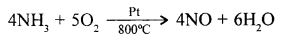Question 4.
The following questions are based on the preparation of ammonia gas in the laboratory:

1. Explain why ammonium nitrate is not used in the preparation of ammonia.
2. Name the compound normally used as a drying agent during the process.
3. How is ammonia gas collected ? Explain why it is not collected over water.

1. Ammonium nitrate does not undergo a reversible sublimation reaction, it melts and then decompses into nitrogen oxide gas and water vapour. Thus it is not used in the preparation of ammonia.
NH4NO3 → N2O + 2H2O
2. Calcium oxide
3. Ammonia is collected in an inverted dry gas jar by downward displacement of air.
It is highly soluble in water and hence cannot be collected by downward displacement of water.

2013

Question 1.
State one appropriate observation for :
Excess of chlorine gas is reacted with ammonia gas.
A yellow explosive liquid (Nitrogen trichloride) is formed.

Question 2.
Nitrogen gas can be obtained by heating :

(a) Ammonium nitrate
(b) Ammonium nitrite
(c) Magnesium nitric
(d) Ammonium chloride

(b) Ammonium nitrite

Question 3.
State two relevant observations for :
Ammonium hydroxide solution is added to zinc nitrate solution in minimum quantities and then in excess.
A white gelatin like precipitate of zinc hydroxide is formed which dissolves in excess of ammonium hydroxide.

Question 4.
Give balanced equations for :
Reduction of hot Copper (II) oxide to copper using ammonia gas.Question 5.
Copy and complete the following table relating to im¬portant industrial process :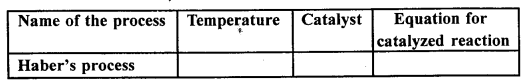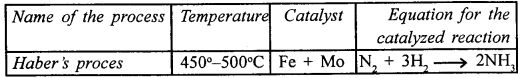Question 6.
Identify : An alkaline gas which produces dense white fumes when reacted with HCl gas.
Ammonia gas.

2014

Question 1.
Fill in the blank from the choices given in bracket : Ammonia gas is collected by _____ (upward displacement of air, a downward displacement of water, a downward displacement of air)
Ammonia gas is collected by a downward displacement of air.

Question 2.
Write balanced equation for : Action of warm water on magnesium nitride.
Action of warm water on magnesium nitride.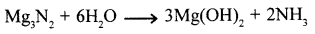Question 3.
Distinguish between the following pairs of compounds using the test given within bracket :

1. Iron(II)sulphate and iron (III) sulphate (using ammonium hydroxide)
2. A lead salt and a zinc salt (using excess ammonium hydroxide)Question 4.
State your observation : Calcium hydroxide is heated with ammonium chloride crystals.ammonia gas is evolved which fumes strongly in moist air.

Question 5.
Name the other ion formed when ammonia dissolves in water. Give one test that can be used to detect the presence of the ion produced.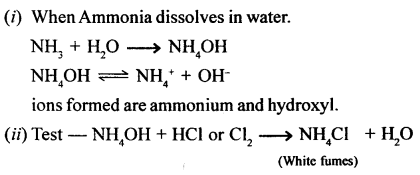Question 6.
State the conditions required for : Catalytic oxidation of ammonia to nitric oxide.
Catalytic oxidation of ammonia to nitric oxide.Conditions for catalytic oxidation of ammonia to nitric oxide : Platinum catalyst and 800°C temperature.

2015

Question 1.
From the list the gases — Ammonia, ethane, hydrogen chloride, hydrogen sulphide, ethyne
Select the gas which is used as a reducing agent in reducing copper oxide to copper.
Hydrogen

Question 2.
State one relevant observation — Ammonia gas is burnt in an atmosphere of excess oxygen.
Ammonia gas bums to form nitrogen gas and steamy fumes of water.

Question 3.
A metal ‘X’ has valency 2 and a non-metal ‘Y’ has a valency 3.
If ‘Y ’ is a diatomic gas, write an equation for the direct combination of X and Y to from a compound.
The equation for the direct combination of X and Y to form a compound isQuestion 4.
Give balanced chemical equations —

1. Lab. preparation of ammonia using an ammonium salt.
2. Reaction of ammonia with excess chlorine.
3. Reaction of ammonia with sulphuric acid.

1. Ammonia is prepared in the laboratory by using ammonium chloride.2. When ammonia reacts with excess of chlorine, it forms nitrogen trichloride and HCl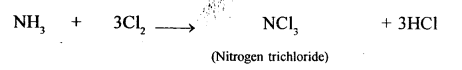3. Ammonia reacts with sulphuric acid to form ammonium sulphate.2016

Question 1.
Write balanced equations for :

1. Action of warm water on AIN.
2. Excess of ammonia is treated with chlorine.
3. An equation to illustrate the reducing nature of ammonia.Question 2.
Name the gas evolved when the following mixtures are heated :

1. Calcium hydroxide and Ammonium chloride.
2. Sodium nitrite and Ammonium chloride.

1. Ammonia gas
2. Nitrogen gas

2017

Question 1.
Write the balanced chemical equation for each of the following –

1. Reaction of ammonia with heated copper oxide.
2. Laboratory preparation of ammonia from ammonium chloride.Question 2.
State one relevant observation for the following reaction – Burning of ammonia in air.
Ammonia bums with a yellowish green flame.

Question 3.
Certain blanks spaces are left in the following tables as C, D & E. Identify each of them.C : Reactants are Magnesium nitride(Mg3N2) and water(H2O).
D : Drying agent is anhydrous calcium oxide (Quick lime).
E : NH3 gas is collected by the downward displacement of air.

Question 4.
Give a balanced chemical equation for each of the following –

1. Catalytic oxidation of ammonia.
2. Reaction of ammonia with nitric acid.Question 1.
State why nitrogenous matter produces ammonia. State a liquid source of ammonia.
When nitrogenous matter (such as animal and vegetable protiens) decays in the absence of air, the putrefying bacteria on the organic matter in the soil or ammonifying bacteria in organic matter produces ammonia.
The liquid souce of ammonia is decaying urine of animals.

Question 2.
Give the word equation and balanced molecular equation for the laboratory preparation of ammonia from NH4Cl and calcium hydroxide.
Ammonium Chloride + Calcium Hydroxide → Calcium Chloride + Water + Ammonia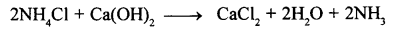Question 3.
Convert ammonium sulphate to ammonia using two different alkalis.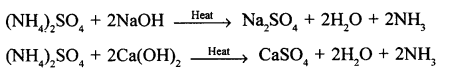Question 4.
State why ammonia is not obtained in the laboratory from NH4NO3 and NaOH.
Ammonium nitrate on heating decomposes explosively with the formation of nitrous oxide and water.Question 5.
State the method used with reasons for drying and collecting ammonia gas.
Calcium oxide (quick lime) is used for drying ammonia. It is because, calcium oxide being basic in nature does not react chemically with ammonia.
CaO + H2O → Ca(OH)2
Chemicals such as CaCl2 (anhydrous), P2O5 and cone H2SO4 are not used for drying ammonia, because they react chemically with it as shown in the equation below :Ammonia is collected by downward displacement of air. Ammonia gas is highly soluble in water, as such it cannot be collected over water. Further, ammonia is lighter than air. As such ammonia is collected by downward displacement of air.

Question 6.
State how you would convert (i) Mg (ii) Ca (iii) Al – to ammonia.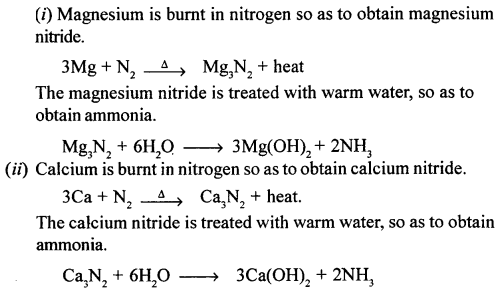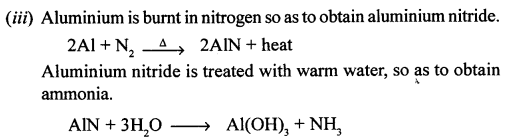Question 7.
Give a balanced equation with all conditions to obtain NH3 from N2 and H2.Conditions for maximum yield of ammonia :

• Pressure : 200-900°C (High pressure)
• Temperature : 450-500°C (Optimum temperature)
• Catalyst : Finely divided iron, Fe
• Promotor : Molybdenum, Mo

Question 8.
State two physical properties of NH3 which enable separation of NH3 from a mixture of NH3, N2 and H2.

1. Ammonia liquifies at a pressure 8 atmosphere at -33°C, but not hydrogen and nitrogen.
2. Ammonia is extremely soluble in water, but not hydrogen and nitrogen.

Question 9.
Compare the density of ammonia with that of air. Name two gases lighter than ammonia.
The vapour density of ammonia is 8.5 and that of air is 14.4. The two gases lighter than ammonia are

1. hydrogen
2. helium.

Question 10.
‘Ammonia is highly soluble in water’. Name two other gases showing similar solubility.
The other highly soluble gases in water are :

1. Hydrogen chloride
2. Sulphur trioxide.

Question 11.
Name the experiment and state its procedure to demonstrate the high solubility of ammonia.
Highly solubility of ammonia can be shown by Fountain Experiment.
To demonstrate the high solubility of ammonia gas in water.Apparatus :

1. Round bottomed flask filled with ammonia gas.
2. Mouth of the flask with a rubber stopper with two holes, one for jet tube and other for dropper containing water.
3. Trough below contains red litmus solution.

Procedure :

1. The dropper containing water is squeezed and few drops of water enters the flask.
2. Ammonia gas present in the flask gets dissolved in water due to its high solubility, which creates a partial vacuum in the flask.
3. Since outside pressure is higher, so red litmus solution rush up the jet tube and emerge as a fountain. (Ammonia gas being basic changed red litmus blue.)
Ammonia gas is lighter than air, hence it is collected by downward displacement of air.
4. Easily liquified at low temperatures.
5. Liquid ammonia boils at – 33.5°C
6. Solid ammonia melts at – 77.5°C

Question 12.
Give an equation for the burning of ammonia in oxygen. State the observation seen.
Ammonia bums in the atmosphere of oxygen with a pale blue flame, forming nitrogen gas and water vapour.Question 13.
Convert ammonia to nitric oxide by catalytic oxidation of ammonia. State all conditions.
When a mixture of 2 (vols.) of oxygen and l(vols.) of ammonia is passed over platinum gauze maintained at 800°C, it reacts to form nitric oxide and water vapour.• Conditions for the reaction : Ostwald’s process
• Temperature : 800°C
• Catalyst : Platinum (Pt)

Question 14.
Draw a simple diagram for the catalytic oxidation of ammonia in the laboratory.Question 15.
Give reasons for the observation seen during catalytic oxidation of ammonia.
The colourless nitric oxide formed undergoes further oxidation to give reddish brown vapours of nitrogen oxide.
The platinum(catalyst) continues to emit a reddish glow even after the heating is discontinued since the catalytic oxidation of ammonia is an exothermic reaction.

Question 16.
Name an industrial process which involves ammonia, oxygen and a catalyst as its starting reactants.
The industrial process is called Ostwald’s process for preparing nitric acid.

Question 17.
State what an aqueous solution of NH3 is called. State how it is prepared giving reasons.
The aqueous solution of ammonia is chemically ammonium hydroxide(NH4OH) (Liquor Ammonia).
It is prepared by connecting the delivery tube of the apparatus generating ammonia with an inverted funnel whose rim is just dipping in water contained in beaker.
This arrangement provides

1. large surface area for the absorption of ammonia and
2. prevents back suction
NH3 + H2O → NH4 OH

Question 18.
State why an aq. soln. of NH3

1. turns red litmus blue
2. is a weak base and a weak electrolyte.

1. Ammonia on dissolving in water furnishes ammonium (NH4+) ions and hydroxyl (OH) ions.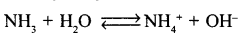The presence of OH turns the red litmus blue.
2. When ammonia dissolves in water, it forms ammonium hydroxide. The ammonium hydroxide dissociates to NH4+ ions and OH ions.
However, the degree of dissociation of ammonium hydroxide molecules is very low. Thus, due to the presence of few OH- ions it is a weak base, as well as weak electrolyte.

Question 19.
State two different methods of preparing NH4Cl using hydrochloric acid.

1. When ammonium hydroxide is treated with hydrochloric acid, neutrilisation reaction takes place with formation of Ammonium chloride
NH4OH + HCl (dil.) → NH4Cl + H2O
2. When ammounium carbonate is treated with hydrochloric acid, it forms ammonium chloride, carbon dioxide and water.
(NH4)2 CO3 + 2HCl (dil.) → 2NH4Cl + CO2 + H2O

Question 20.
Convert (i) ammonia (ii) ammonium hydroxide to an ammonium salt using (a) HNO3 (b) H2SO4.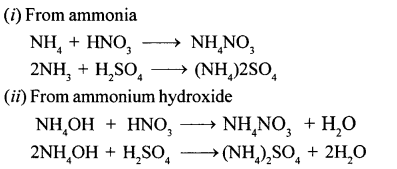Question 21.
State a reason why reaction of liquor ammonia with nitric acid is a neutralization reaction.
Liquor ammonia is a saturated solution of ammonia in water. Ammonia, NH3 dissolves in water to give ammonium hydroxide which dissociates partially to give NH4+ and OH ions. Due to presence of OH ions, ammonium hydroxide acts as an alkali.The reaction between an acid (nitric acid) and an alkali or base is a neutralisation reaction.Hence, the reaction between liquor ammonia and nitric acid is a neutralisation reaction.

Question 22.
State why an aqueous solution of ammonia (NH4OH) is used for identifying cations.
Because of its colour and solubility in excess NH4OH.

Question 23.
State how NH4OH is used for identify :

1. Fe2+
2. Fe3+
3. pb2+
4. Zn2+
5. Cu2+ cations.

Give also a balanced equation in each case for a known example.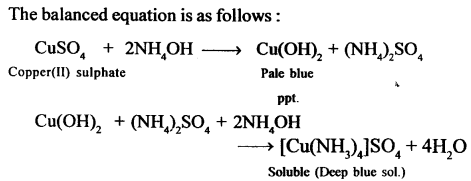Question 24.
State why the blue ppt. formed on addition of NH4OH to CuSO4 soln. dissolves to give a deep blue solution with excess of NH4OH. Give an equation for the reaction. State why Zn(OH)2 is soluble in excess of NH4OH.
CuSO4 when reacts with excess of ammonia it produces needle shape crystals of tetraammine copper(II) sulphate complex which possess a beautiful deep blue colour.Question 25.
Give balanced equations for the reducing reactions of ammonia with
(i) copper (II) oxide, (ii) lead (II) oxide, (iii) chlorine using, (a) excess NH3(b) excess Cl2.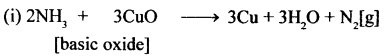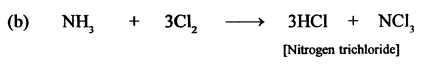Question 26.
State five tests for ammonia where a colour change is involved.
Tests for ammonia :

1. Ammonia turns red (or purple) litmus solution to blue.
2. Ammonia turns methyl orange solution to yellow.
3. Ammonia turns phenolphthalein (colourless) solution to pink.
4. Ammonia turns Nesseler’ reagent (colourless) solution to pale brown.
5. Ammonia (when in excess) gives a deep blue coloured solution with CuSO4 (aq.)

Question 27.
State (i) a light neutral gas (ii) an acid (iii) an explosive (iv) a fertilizer — obtained from ammonia.

1. Hydrogen
2. Nitric acid
3. NH4NO3
4. Ammonium sulphate.

Question 28.
Name an ammonium salt which is a constituent of (a) smelling salts (b) dry cells.
Give reasons for the use of the named ammonium salt for the same.

(a) Smelling salts : Ammonium carbonate, (NH4)2CO3 is used as smelling salt. It is an unstable white solid decomposes to give pungent smellingNH3 gas.
(NH4)2CO3 → 2NH3 + CO2 + H2O
The pungent smelling NH3 gas revives a fainted person. (NH4)2CO3 is always kept in a tightly closed container to prevent it from decomposition.
(b) Dry cells : Ammonium chloride(NH4Cl) is used in dry cells. It oxidises Zn to Zn2+ ions. The electrons so produced constitute the electric current.

Question 29.
Give one use with reason of

1. an aqueous solution of NH3
2. liquefied NH3.

(i) Use of an Aqueous solution of Ammonia (NH3) : An aqueous solution of NH3 is called liquor ammonia. Being a base, it can easily emulsify oils and fats. Therefore, it is used for removing oil and fat stains from clothes carpets, upholstery etc. It is also used for cleaning window panes, porcelain articles etc.
(ii) Use of Liqueified Ammonia (NH3) : Liquid ammonia is used as refrigerant i.e. for producing low temperature. This is due to the following reasons:

(a) It is highly volatile.
(b) It can be easily liquefied under high pressure and low temperature.
(c) It’s latent heat of evaporation is very high.

Question 30.
State what are chlorofluorocarbons and give their use. Give a reason why they are ozone depleting. State a suitable alternative to chlorofluorocarbons which are non-ozone depleting.
Chlorofluorocarbons (CFC’s) are compounds of carbon with chlorine or fluorine.
CFC are chemicals which like liquefied ammonia gas are used in refrigeration gas. They are also used as coolants in refrigeration and A/c Plants and aerosol sprays and cleansing agents.
These CFC’s deplete ozone layer and also contribute to global warming. Thus these CFC’s are harmful to life. The CFC’s are decomposed by ultraviolet rays coming from sun to produce highly reactive chlorine atoms i.e. free Cl radicals.
Suitable alternatives to chloroflurocarbons which are not depleting ozone are :
HCFC – Hydrochloroflurocarbons; and HFC – 125 Hydrochloroflurocarbons ; which act as a substitute for CFCs, which are non-ozone depleting.

Unit Test Paper 7b —Ammonia

Q.1. Choose the letter corresponding to the correct answer from — A : NO2, B : NO, C : N2, D : N2O.
The gas obtained when –
1. Dry ammonia and dry oxygen gas are ignited together.
2. Ammonia is passed over heated litharge.
3. A greenish yellow gas reacts with excess ammonia.
4. (a) Dry NH3 and O2 are passed over heated Pt.
(b) The gaseous product obtained is further oxidised.
5. Ammonium nitrite undergoes thermal decomposition.

1. C(N2)
2. C(N2)
3. C(N2)
4. (a) B(NO),(b) A(NO2)
5. D(N2O)

Q.2. State the colour of :

Question 1.
Phenolphthalein solution after passage of ammonia through it.
Colourless phenolphthalein changes to pink colour.

Question 2.
Copper (II) hydroxide solution after-addition of ammonium hydroxide in excess to it.
The pale blue precipitate turns into a deep blue coloured solution.

Question 3.
The flame obtained on burning dry ammonia in oxygen.
Greenish yellow flame.

Question 4.
The solution obtained on addition of excess ammonium hydroxide to zinc sulphate solution.
White gelantinous precipitate dissolves.

Question 5.
The vapours obtained when ammonia-oxygen gas mixture is passed over heated Pt.
The colourless NO gas first produced is further oxidises to brown coloured NO2.

Q.3. Give balanced equations for the following conversions — A, B, C, D and E.Q.4. Give reasons for the following.

1. An aqueous solution of ammonia acts as a weak base.
2. A mixture of ammonium nitrate and slaked lime are not used in the lab. preparation of ammonia gas.
3. Finely divided iron catalyst does not affect the percentage yield of ammonia in Haber’s process.
4. Ammonium salts are formed when ammonia reacts with dilute acids in the gaseous or aq. medium.
5. Aqueous solution of lead and zinc nitrate can be distinguished using an aqueous solution of ammonia.

1. Ammonia molecule contains a lone pair of electrons on N- atom, which accepts a proton from water, and forms ammonium ion and hydroxide ion, this makes ammonia solution alkaline and thus, it acts as a base.
NH3+ H2O → NH4+ + OH
However, as the concentration of the hydroxyl ions that are furnished is less, therefore aqueous solution of ammonia acts as a weak base.
2. Because ammonium nitrate is explosive and decomposes itself.
3. Formation of NH3 from N2 and H2 in haber’s process is a reversible reaction. In a reversible reaction, a catalyst does not increase the yield of the product. It only helps in attaining the equilibrium in a shorter time.4. Ammonia in gaseous form neutralises acids to form salts.
5. Zinc nitrate forms white gelatinous ppt. while lead nitrate forms chalky white ppt.

Q.5. Complete the statements by selecting the correct word from the words in brackets.

1. The salt solution which does not give an insoluble precipitate on addition of ammonium hydroxide in small amount is _____ (Mg(NO3)2 / NaNO3/Cu(NO3)2]
2. The alkaline behaviour of liquor ammonia is due to the presence of _____ ions, (ammonium / hydronium / hydroxyl)
3. Ammonia in the liquefied form is _____ (acidic / basic / neutral)
4. Ammonia reduces chlorine to _____ (nitrogen / hydrogen chloride / ammonium chloride)
5. The chemical not responsible for ozone depletion is _____ [methyl chloride / ammonia / chloroflourocarbons]

1. NaNO3
2. Hydroxyl
3. basic
4. Ammonium chloride .
5. Ammonia

Q.6. Select the most probable substance from A, B, C, D and E which need to be added to distinguish :